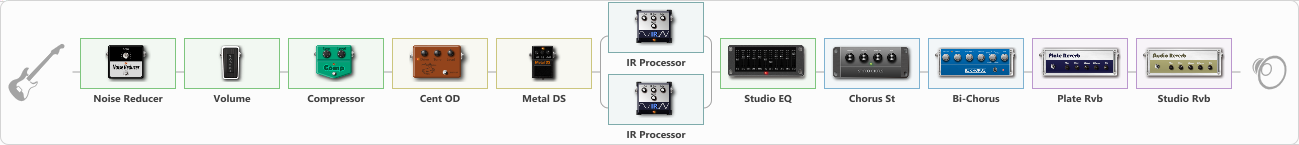Discussion in 'ToneLib-GFX presets' started by Neronte, Oct 30, 2022.

Preset name: Rockman X-100

Effects chain:Effect: "Noise Reducer" (Dynamics / Filter), active - "yes"
{
"Sens" = 75
"Mode" = Hard
}

Effect: "Volume" (Dynamics / Filter), active - "yes"
{
"Pedal Pos." = 25
"Pan" = 0
}

Effect: "Compressor" (Dynamics / Filter), active - "yes"
{
"Sense" = 1
"Level" = 100
}

Effect: "Cent OD" (Overdrive / Distortion), active - "yes"
{
"Drive" = 35
"Tone" = 0
"Level" = 35
}

Effect: "Metal DS" (Overdrive / Distortion), active - "yes"
{
"Dist" = 100
"Bass" = 85
"Middle" = 40
"Treble" = 40
"Level" = 75
}

Effect: "Splitter" (Dynamics / Filter)
{
"A-Bypass" = Off
"A-Pan" = -50
"A-Level" = 55
"B-Bypass" = Off
"B-Pan" = 53
"B-Level" = 55
"Width" = 0

'A' branch:
{

Effect: "IR Processor" (Cabinets), active - "yes"
{
"IR" = ROCKMAN_IR
"Low Cut (Hz)" = 0
"Hi Cut (kHz)" = 4.5
"Mix" = 100
"Level (dB)" = 0
}
}
'B' branch:
{

Effect: "IR Processor" (Cabinets), active - "yes"
{
"IR" = ROCKMAN_IR
"Low Cut (Hz)" = 0
"Hi Cut (kHz)" = 4.5
"Mix" = 100
"Level (dB)" = 0
}
}
}

Effect: "Studio EQ" (Dynamics / Filter), active - "yes"
{
"31 Hz" = -15
"62 Hz" = -5
"125 Hz" = 0
"250 Hz" = 0
"500 Hz" = 2
"1 kHz" = 0
"2 kHz" = 0
"4 kHz" = 0
"8 kHz" = 0
"16 kHz" = -15
"Level (dB)" = 5
}

Effect: "Chorus St" (Modulation / Sfx), active - "yes"
{
"Speed" = 1.9
"Depth" = 31
"Center" = 4.4
"Mix" = 35
}

Effect: "Bi-Chorus" (Modulation / Sfx), active - "yes"
{
"Speed A" = 3.2
"Speed B" = 4.0
"Depth" = 25
"Reso" = 0
"Mode" = Parallel
"Mix" = 25
}

Effect: "Plate Rvb" (Reverberation), active - "yes"
{
"Time" = 1.0
"PreDelay" = 30
"LoDamp" = 17
"HiDamp" = 25
"Mix" = 50
}

Effect: "Studio Rvb" (Reverberation), active - "yes"
{
"Time" = 1.0
"PreDelay" = 32
"LoDamp" = 0
"HiDamp" = 12
"Mix" = 25
}

Note: You will need to download and install the ToneLib-GFX software to use the preset.